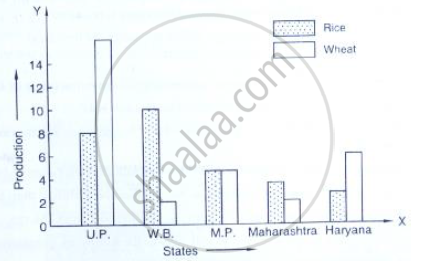# Read the Following Bar Graph and Answer the Following Questions:(I) What Information is Given by the Bar Graph? (Ii) Which State is the Largest Producer of Rice? - Mathematics(i) What information is given by the bar graph?
(ii) Which state is the largest producer of rice?
(iii) Which state is the largest producer of wheat?
(iv) Which state has total production of rice and wheat as its maximum?
(v) Which state has the total production of wheat and rice minimum?

#### Solution

(1) The bar graph represents the production of rice and wheat in different states of India.

(2) According to the height of the bars corresponding to rice, W.B. is the largest producer of rice.

(3) According to the height of the bars corresponding to wheat, U.P. is the largest producer of wheat.

(4) U.P. has the maximum total production of rice and wheat, which is 8 + 16 = 24 units

(5) Maharashtra has the minimum total production of rice and wheat, which are exactly 2 + 4 = 6 units.

Concept: Graphical Representation of Data
Is there an error in this question or solution?

#### APPEARS IN

RD Sharma Mathematics for Class 9
Chapter 23 Graphical Representation of Statistical Data
Exercise 23.1 | Q 9 | Page 11

Share